# Excel Review Excel review Excel is a spreadsheet

• Slides: 10Excel Review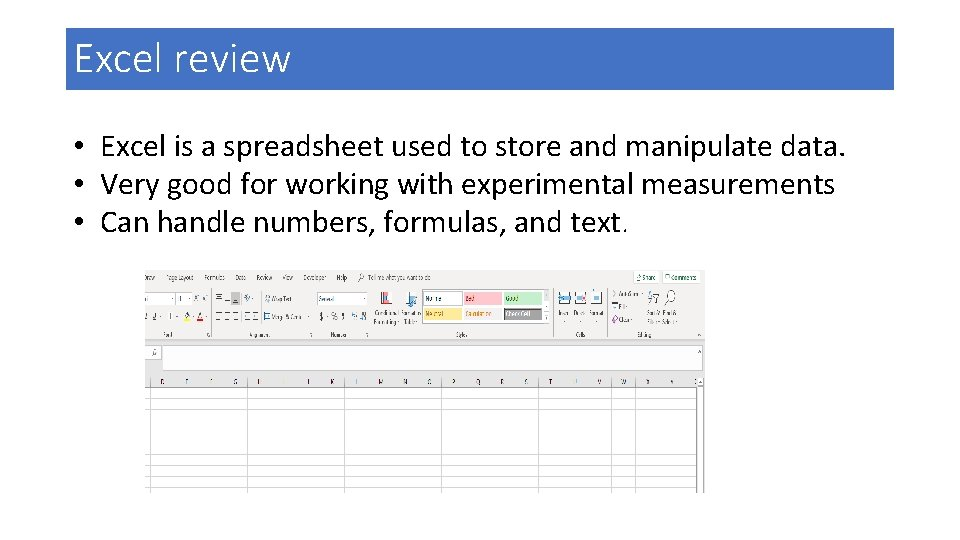Excel review • Excel is a spreadsheet used to store and manipulate data. • Very good for working with experimental measurements • Can handle numbers, formulas, and text.Excel review tabs commands column N ribbon Name box formula bar scroll bar spreadsheet Row 11 cell N 11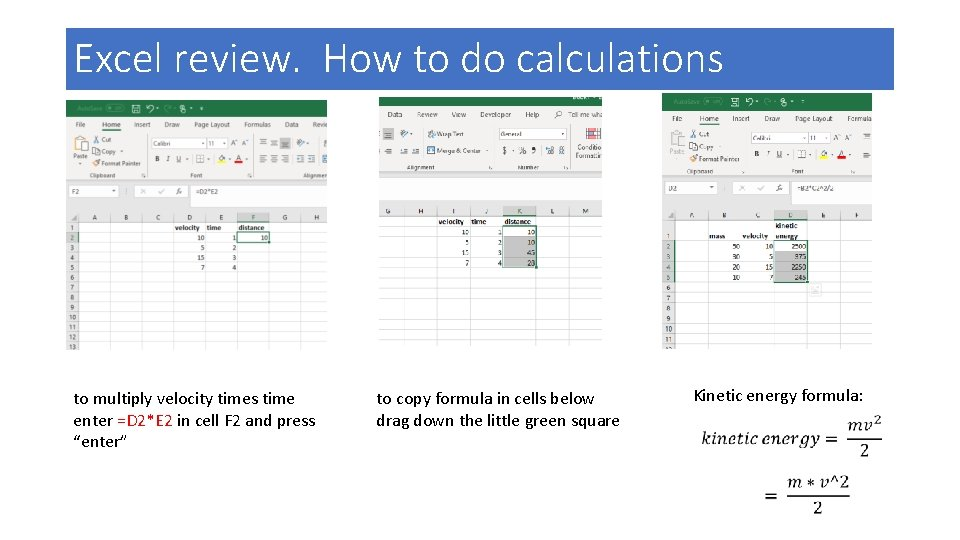Excel review. How to do calculations to multiply velocity times time enter =D 2*E 2 in cell F 2 and press “enter” to copy formula in cells below drag down the little green square Kinetic energy formula:Excel review. Plotting EXERCISE Plot Y vs. X • Y vs. X means Y will be plotted on the vertical axis and X on the horizontal axis • Ensure X is to the left of Y • Select all data in columns B and C • Select the “Insert” tab • In the “Charts” section select the scattered plot. This opens up a window containing different types of plots. • Select the dotted plot (top left) The plot shown on the left will be createdExcel review. Plotting EXERCISE Get equation for plot • Right click on one of the dots of the plot. A window will open up • Select “Format Trendline”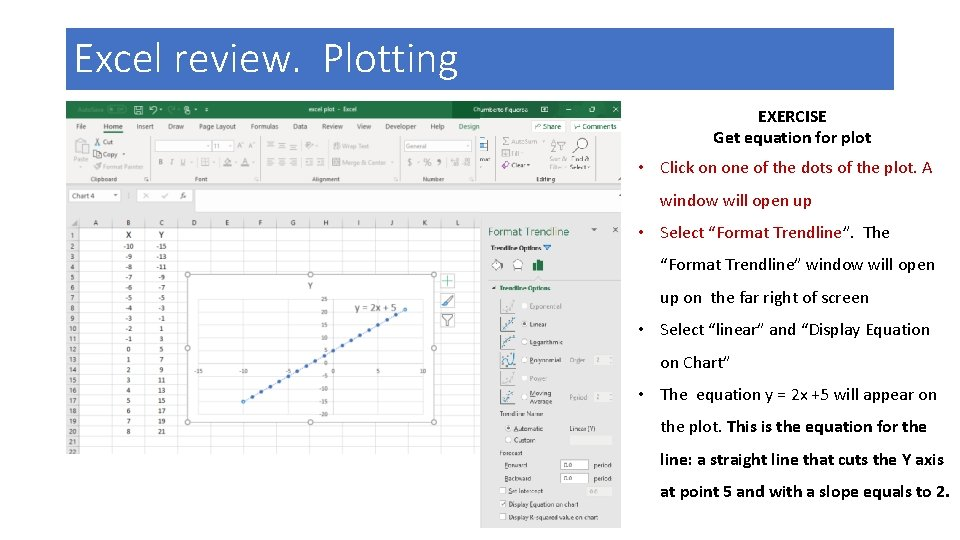Excel review. Plotting EXERCISE Get equation for plot • Click on one of the dots of the plot. A window will open up • Select “Format Trendline”. The “Format Trendline” window will open up on the far right of screen • Select “linear” and “Display Equation on Chart” • The equation y = 2 x +5 will appear on the plot. This is the equation for the line: a straight line that cuts the Y axis at point 5 and with a slope equals to 2.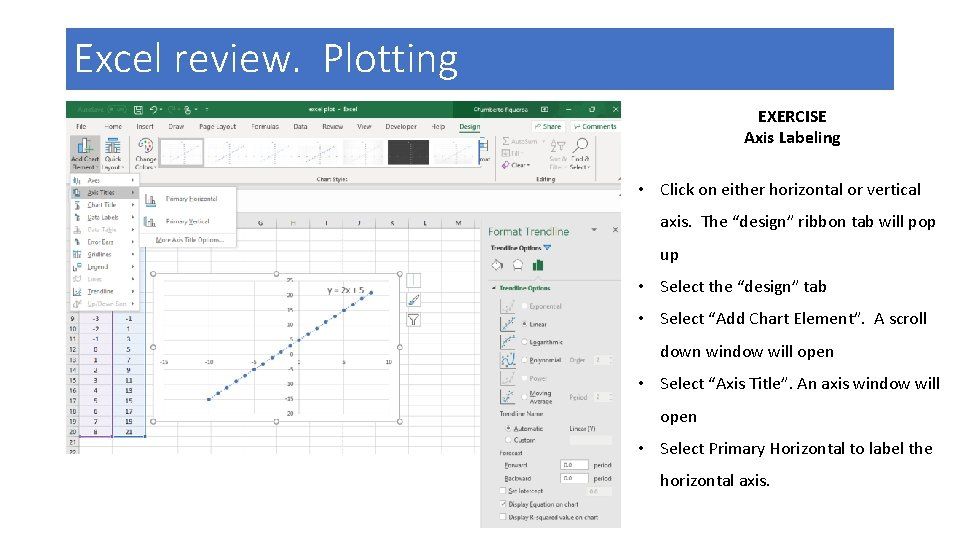Excel review. Plotting EXERCISE Axis Labeling • Click on either horizontal or vertical axis. The “design” ribbon tab will pop up • Select the “design” tab • Select “Add Chart Element”. A scroll down window will open • Select “Axis Title”. An axis window will open • Select Primary Horizontal to label the horizontal axis.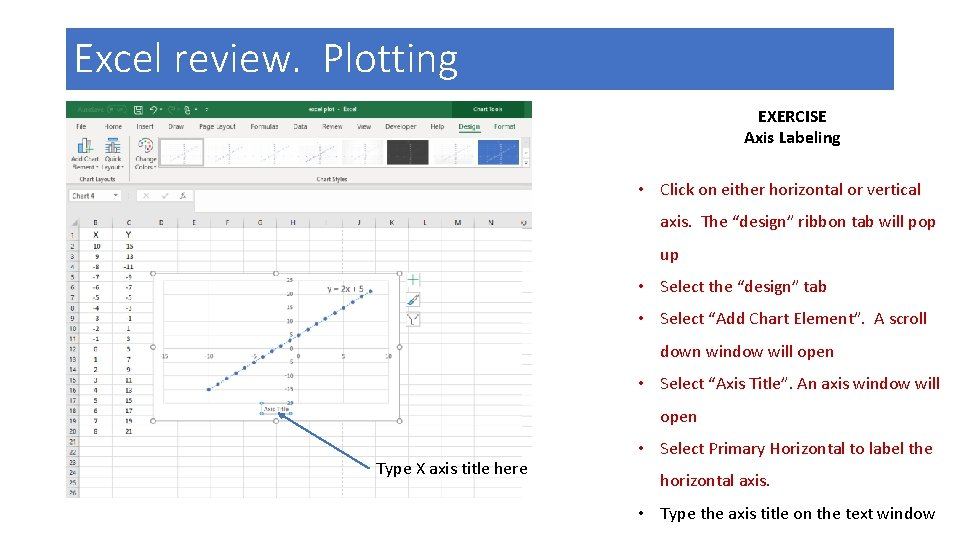Excel review. Plotting EXERCISE Axis Labeling • Click on either horizontal or vertical axis. The “design” ribbon tab will pop up • Select the “design” tab • Select “Add Chart Element”. A scroll down window will open • Select “Axis Title”. An axis window will open Type X axis title here • Select Primary Horizontal to label the horizontal axis. • Type the axis title on the text window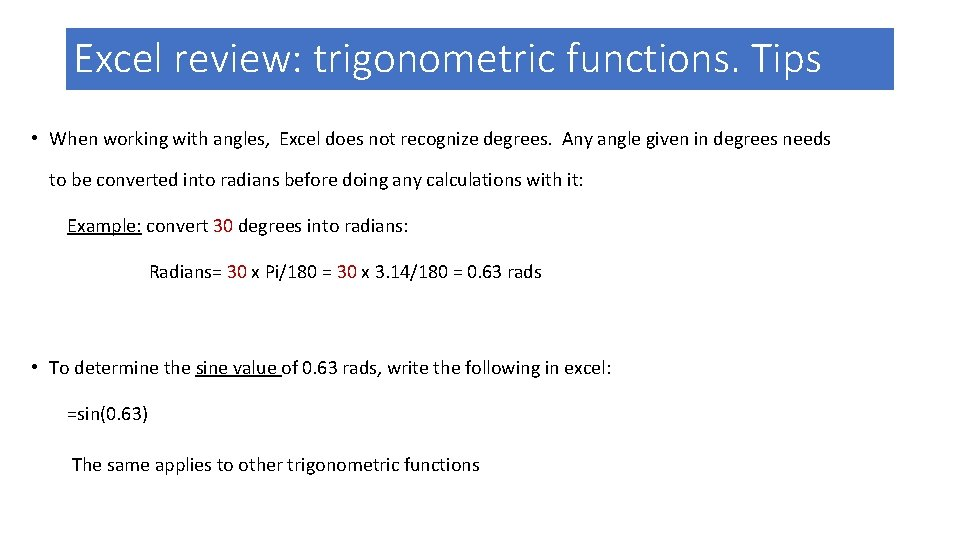Excel review: trigonometric functions. Tips • When working with angles, Excel does not recognize degrees. Any angle given in degrees needs to be converted into radians before doing any calculations with it: Example: convert 30 degrees into radians: Radians= 30 x Pi/180 = 30 x 3. 14/180 = 0. 63 rads • To determine the sine value of 0. 63 rads, write the following in excel: =sin(0. 63) The same applies to other trigonometric functions